# Why is simple harmonic motion so important?

Oscillations are happening all around us, from the beating of the human heart, to the vibrating atoms that make up everything. Simple harmonic motion is a very important type of periodic oscillation where the acceleration (α) is proportional to the displacement (x) from equilibrium, in the direction of the equilibrium position.

###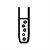What are frequency and period?

Since simple harmonic motion is a periodic oscillation, we can measure its period (the time it takes for one oscillation) and therefore determine its frequency (the number of oscillations per unit time, or the inverse of the period).

The two most common experiments that demonstrate this are:

1. Pendulum - Where a mass m attached to the end of a pendulum of length l, will oscillate with a period (T). Described by: T = 2π√(l/g), where g is the gravitational acceleration.

2. Mass on a spring - Where a mass m attached to a spring with spring constant k, will oscillate with a period (T). Described by: T = 2π√(m/k).

By timing the duration of one complete oscillation we can determine the period and hence the frequency. Note that in the case of the pendulum, the period is independent of the mass, whilst the case of the mass on a spring, the period is independent of the length of spring. The period of a simple harmonic oscillator is also independent of its amplitude.

From its definition, the acceleration, a, of an object in simple harmonic motion is proportional to its displacement, x: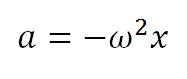where ω is the angular frequency and can be determined either by knowing the period (ω = 2π/T) or the frequency (ω = 2πf). Recalling that velocity (v) is the time derivative of distance and acceleration is the time derivative of velocity, it can be shown that when starting from the amplitude (A), the solution follows a sinosuidal function of the form x = A cos(ωt)

The displacement against time would then look something like this: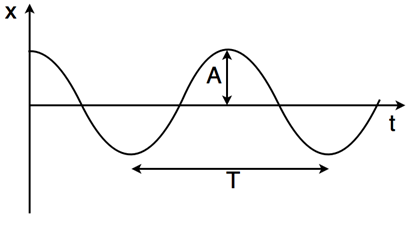With the velocity and acceleration graphs given by the time derivatives. These oscillators also demonstrate the transfer between kinetic and potential energy. At the maximum displacement, all the energy in system is in the form of potential energy and the velocity is zero, but this is all converted into kinetic energy once the mass reaches the equilibrium position where it has maximum velocity.

###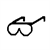How accurate can our measurements be?The experiments described here demonstrate the use of a mix of analogue and digital apparatus to measure quantities including mass, length and time. In this experiment one of the major sources of error is down to the human reaction time when measuring the period. To improve the accuracy on the period, the timings can be taken over multiple oscillations and by averaging over several measurements of the period. To obtain more accurate measurements of the spring constant and the gravitational acceleration, repeated measurements should be taken using various pendulum lengths and masses.

Also, measuring period over a longer time frame (and hence over multiple oscillations) will increase the accuracy since the human error will be a smaller fraction of the recorded time. It can also be useful to use a pin or tag to act as a fiduciary marker showing the equilibrium position. Assuming simple harmonic motion, the periodic nature of these systems mean that there should be no excuse when it comes to taking multiple measurements!

###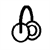Laboratory Confessions

In the Laboratory Confessions podcast researchers talk about their laboratory experiences in the context of A Level practical assessments. In this episode we look at generating and measuring waves and the use of appropriate digital instruments.

###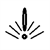What do your measurements mean?

The vibrations and oscillations that surround us in our everyday lives are generally much more complicated than those we encounter in simple harmonic motion. This means that effects such as damping, which acts to reduce the amplitude by removing energy from the system, are a good example of where simple harmonic motion contributes to improving our day-to-day lives. Whilst simple harmonic motion is a simplification, it is still a very good approximation.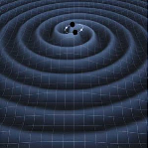Simple harmonic motion is important in research to model oscillations for example in wind turbines and vibrations in car suspensions. At the University of Birmingham, one of the research projects we have been involved in is the detection of gravitational waves at the Laser Interferometer Gravitational-Wave Observatory (LIGO). There the detectors are so sensitive that careful modelling and minimisation of the surrounding vibrations and noise are crucial. Another notable research project is the work of the Birmingham Solar Oscillation Network (BiSON), who focus on measuring oscillations in the sun (helioseismology) and nearby stars (astroseismology) to learn about their internal structures.

###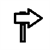Next steps...

These links are being provided as a convenience and for informational purposes only; they do not constitute an endorsement or an approval by the University of Birmingham of any of the information contained on external website. The University of Birmingham bears no responsibility for the accuracy, legality or content of the external site or for that of subsequent links. Please contact the external site for answers to questions regarding its content.